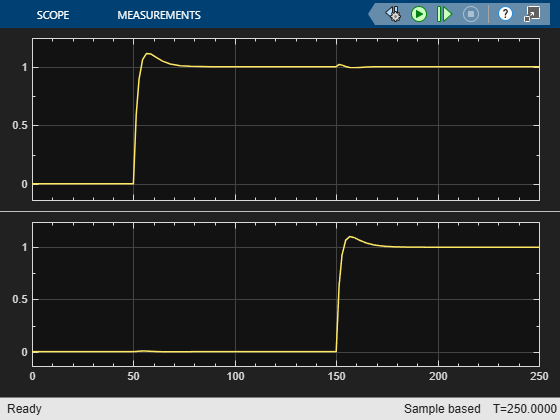## Import LTI Model Objects into Simulink

Use the LTI System block to import linear system model objects into Simulink®. You can simulate linear systems represented as LTI model objects, and incorporate such systems as elements of Simulink models of more complex systems.

In the block parameters, set the LTI system variable parameter to the LTI model to import. For state-space models, set the Initial states parameter to a vector to specify non-zero initial states.

### Simulate LTI Model in Simulink

The `LTISystemBlockSimulation` model shows how to use an LTI System block to simulate the response of a SISO transfer function to a step input.To specify a model for the LTI System block, set the LTI system variable block parameter to either:

• The variable name of an LTI model in the MATLAB® workspace or model workspace, such as `sys`.

• A MATLAB expression that evaluates to an LTI model, such as `tf(1,[1 1])`.

For example, you can specify a state-space (`ss`), zero-pole-gain (`zpk`), or transfer function (`tf`) model. You can simulate SISO models or MIMO models, and continuous-time or discrete-time models.

In `LTISystemBlockSimulation` model, the LTI system variable parameter is a MATLAB expression, `tf(1,[1 2 5])`, which creates a continuous-time SISO transfer function. If the specified system is a state-space (`ss`) model, then you can specify initial state values by setting the Initial states parameter.

Simulate the model, and examine the result in the scope.This example simulates the system response to a step input at t = 2 s. Use the LTI System block to import an LTI model object anywhere in your Simulink model to simulate the linear system response to any input.

### Import MIMO LTI Model into Simulink

This model shows how to use an LTI System block to represent a MIMO linear system in Simulink®.

The LTI System block has one input and one output, even when you specify a MIMO model for the block. In that case, the block input and output become vector signals. For instance, the model `LTISystemBlockMIMO` uses an LTI system block to represent a MIMO plant in a control system.In this model, the LTI System specified in the block is `Gm`, a 2-output, 2-input transfer function model stored in the model workspace. A Mux block combines the two controller outputs into a vector signal for the LTI System block input. Similarly, a Demux block separates the vector output of the LTI System block into two scalar signals.

Simulate the model, and examine the result in the scope.This example simulates a closed-loop system response to a t = 50 s step at the first input and a t = 150 s step at the second input. You can use the LTI system block anywhere you want to insert an LTI system into a Simulink model.